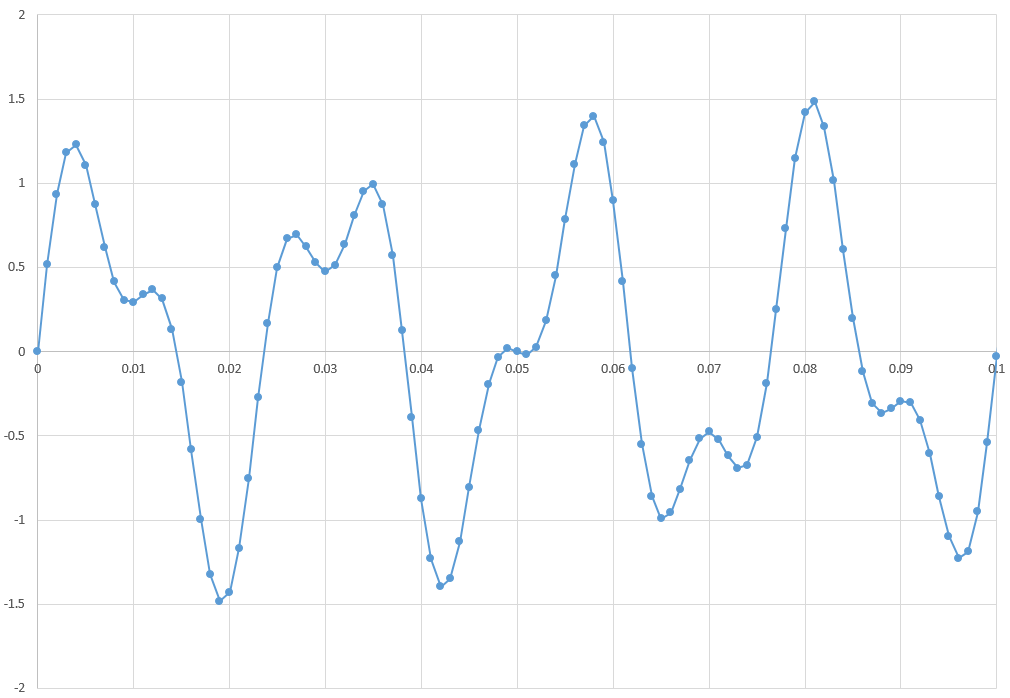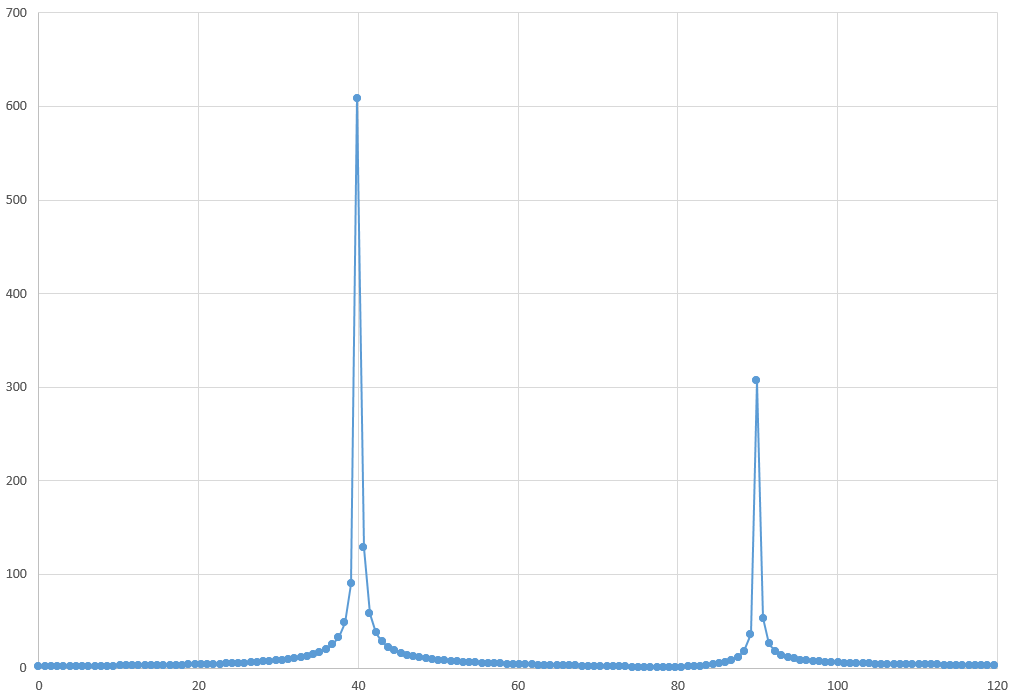The Vertica Forum recently got a makeover! Let us know what you think by filling out this short, anonymous survey.

# Extending Vertica with Python functions: adding NumPy FFT as a UDx

Vertica 9.1 adds User-Defined Transform Function (UDTF) support for Python UDx, allowing you to add a much greater range of existing libraries and functions to Vertica. In this example, I'll add Fast Fourier Transform (FFT) from the NumPy package.

FFT is a way to transform time-domain data into frequency-domain data. My test dataset is a complex sine wave inserted into Vertica as X,Y float coordinates of amplitude versus time. Here's a table extract and graph of the curve:

```=> select * from t limit 5;
time |     amplitude
-------+-------------------
0 |                 0
0.001 | 0.516358837261612
0.002 | 0.933821744458138
0.003 |   1.1803794797964
0.004 |  1.22967657062023
```My UDTF consists of a factory class "pyFFTFactory" that defines the input and output types of my function and returns instances of my function, as well as the class "pyFFT" that actually defines my function, converting input tuples to a format NumPy can work with, running the calculation, and returning the result set as tuples that Vertica can use. See the Python code at https://github.com/bryanherger/vertica-python-fft/blob/master/pyFFT.py

Now I can call my Python code from SQL and store the result set in a new table:
`CREATE TABLE t_fft AS SELECT pyFFT(a, b) OVER() FROM t;`

This produces output of amplitude vs. frequency as X,Y float coordinates shown in the following table extract and graph:

```=> select * from t_fft limit 5;
frequency     |    amplitude
-------------------+------------------
0 | 1.82150253105139
0 | 1.82150253105139
0.781860828772479 | 1.82391772795915
0.781860828772479 | 1.82391772795915
1.56372165754496 |  1.8311636695427
```My original sine wave function, seen in the sample SQL, was y = sin(40 * 2 * 3.14 * x) + 0.5 * sin(90 * 2 * 3.14 * x), reflected here in the transform as (scaled) 1x peak at 40 and a 0.5x peak at 90.

You can find the full example Python and SQL and setup notes at https://github.com/bryanherger/vertica-python-fft

This should apply to many more functions as long as you define correct input and output types and column counts. You can even distribute processing across nodes using partitions in the OVER() clause - something I'll cover in a future blog post.